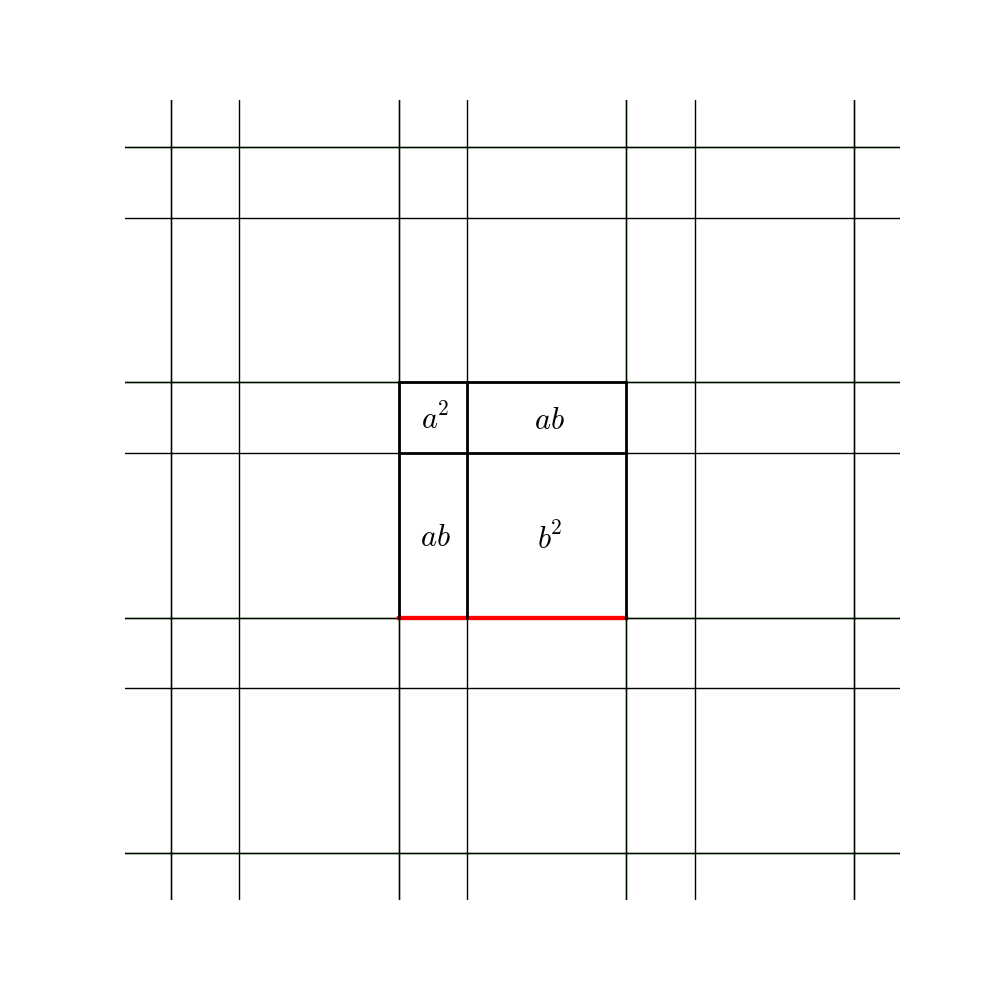## Visualization of the law of cosines

c2 = a2 + b2 - 2ab cos θ

When θ=180, the law of cosines is (a+b)2 = a2 + b2 + 2ab.
When θ=90, the law of cosines is the Pythagorean theorem.Gif © 2018 Linda Brown Westrick